| Next || Previous || Top |

## LLNL_AQUEOUS_MODEL_PARAMETERS

This keyword data block is used to define aqueous model parameters for the Lawrence Livermore National Laboratory aqueous model. The parameters are the temperature-dependent values of the Debye-Hückel A , B , and B -dot parameters and the expression for the activity coefficient of aqueous carbon dioxide as a function of temperature and ionic strength. The LLNL_AQUEOUS_MODEL_PARAMETERS data block is used in the database file llnl.dat , which is the only context in which it can be used.

###### Example data block
`Line 0: LLNL_AQUEOUS_MODEL_PARAMETERS`
`Line 1: -temperature`
`Line 2:	0.0100		25.0000		60.0000		100.0000`
`Line 2a	150.0000		200.0000		250.0000		300.0000`
`Line 3: -dh_a`
`Line 4:	0.4939		0.5114		0.5465		0.5995`
`Line 4a:	0.6855		0.7994		0.9593		1.2180`
`Line 5: -dh_b`
`Line 6:	0.3253		0.3288		0.3346		0.3421`
`Line 6a:	0.3525		0.3639		0.3766		0.3925`
`Line 7: -bdot`
`Line 8:	0.0374		0.0410		0.0438		0.0460`
`Line 8a:	0.0470		0.0470		0.0340		0.0000`
`Line 9: -co2_coefs`
`Line 10:	-1.0312		0.0012806`
`Line 10a:	255.9		0.4445`
`Line 10b:	-0.001606`
###### Explanation

Line 0: LLNL_AQUEOUS_MODEL_PARAMETERS

LLNL_AQUEOUS_MODEL_PARAMETERS is the keyword for the data block. No other data are input on the keyword line.

Line 1: -temperature

-temperature --Begins a block of data that defines a temperature grid. Values of the other parameters are specified at each of the temperatures in the grid. Optionally, temperature , temperatures , or -t [ emperatures ].

Line 2: list_of_temperatures

list_of_temperatures --Temperatures, °C, for the temperature grid. Any number of temperatures can be given, but values of the parameters defined by -dh_a , -dh_b , and -bdot must be defined for each temperature.

Line 3: -dh_a

-dh_a --Begins a block of data that defines the Debye-Hückel A parameter at each temperature in the temperature grid. Optionally, adh , dh_a , -a [ dh ], or -d [ h_a ].

Line 4: dh_a_values

dh_a_values --Values of Debye-Hückel A for each temperature in the temperature grid.

Line 5: -dh_b

-dh_b --Begins a block of data that defines the Debye-Hückel B parameter at each temperature in the temperature grid. Optionally, bdh , dh_b , -b [ dh ], or -dh_b .

Line 6: dh_b_values

dh_b_values --Values of Debye-Hückel B for each temperature in the temperature grid.

Line 7: -bdot

-bdot --Begins a block of data that defines the Debye-Hückel B -dot parameter at each temperature in the temperature grid. Optionally, bdot , b_dot , -bdo [ t ], or -b_ [ dot ].

Line 8: dh_bdot_values

dh_bdot_values --Values of Debye-Hückel B -dot for each temperature in the temperature grid.

Line 9: -co2_coefs

-co2_coefs --Begins a block of data that defines the parameters in the expression for the activity coefficient of CO 2 (aq) as a function of temperature and ionic strength. Optionally, c_co2 , co2_coefs , -c [ _co2 ], or -c [ o2_coefs ].

Line 10: C, F, G, E, H

C, F, G, E, H --Parameters in the expression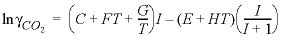.

###### Notes

The Lawrence Livermore National Laboratory aqueous model (Daveler and Wolery, 1992) uses this expression for the log (base 10) of an activity coefficient: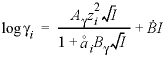, whereis the Debye-Hückel A parameter corresponding to -dh_a ,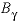is the Debye-Hückel B parameter corresponding to -dh_b ,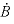is the Debye-Hückel B- dot parameter corresponding to -bdot ,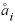is the hard core diameter, which is specific to each aqueous species, and I is the ionic strength. The LLNL_AQUEOUS_MODEL_PARAMETERS data block defines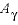,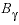, and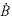as functions of temperature. A temperature grid is defined with -temperatures and values of each of these parameters are specified at each point in the temperature grid. For a given simulation temperature, values of the parameters are interpolated linearly between points on the temperature grid. The ion-specific parameter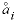is defined in the SOLUTION_SPECIES data block with the identifier -llnl_gamma .

The activity coefficient of aqueous carbon dioxide is defined as a function of ionic strength based on the parameterization of Drummond (1981). The formula for the natural log of the activity coefficient is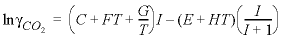, where T is temperature in kelvin; I is ionic strength; and C , F , G , E , and H are defined in order with the -co2_coefs identifier. The activity coefficient calculated from this formula can be applied to specific neutral species by using the identifier -CO2_llnl_gamma in the SOLUTION_SPECIES data block.

###### Example problems

The LLNL_AQUEOUS_MODEL_PARAMETERS data block is used in the llnl.dat database.

###### Related keywords

| Next || Previous || Top |